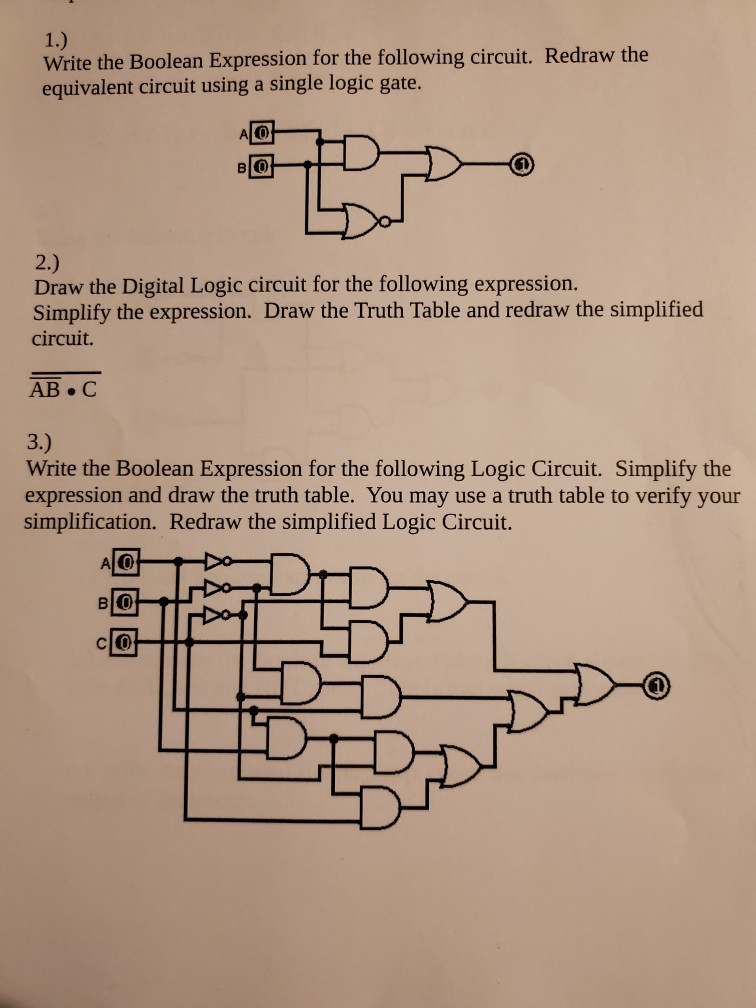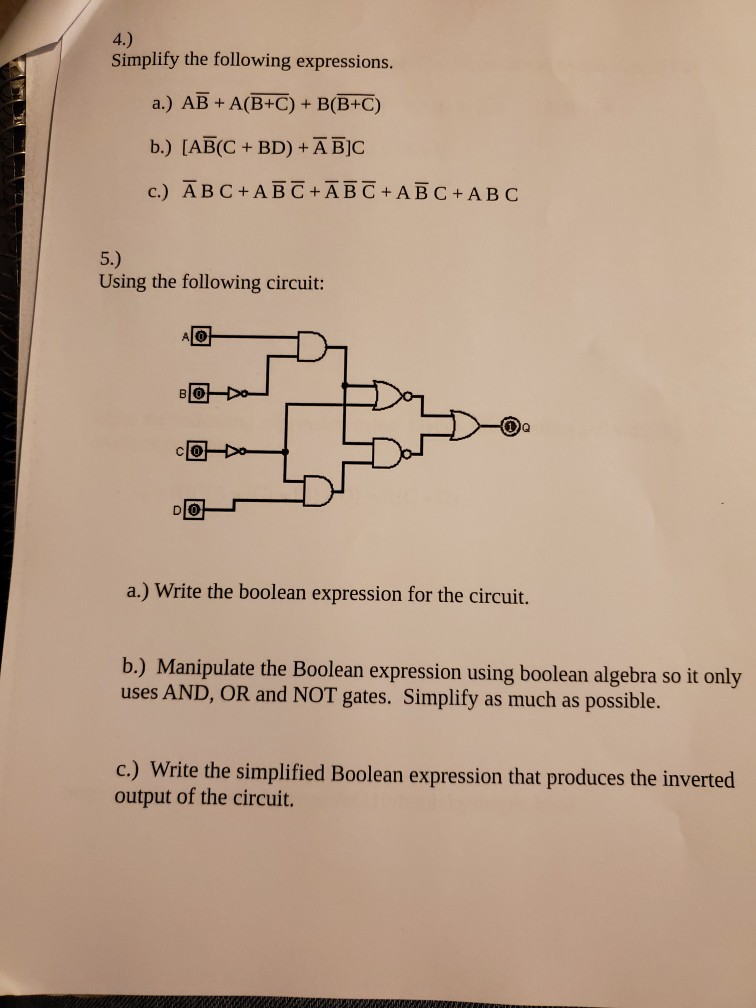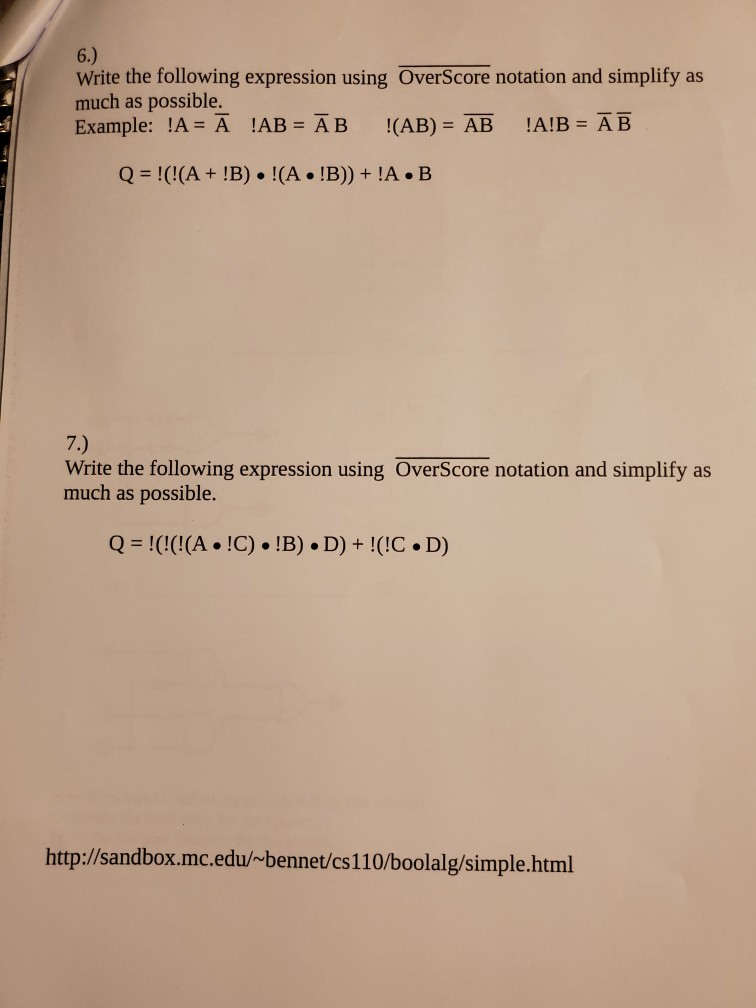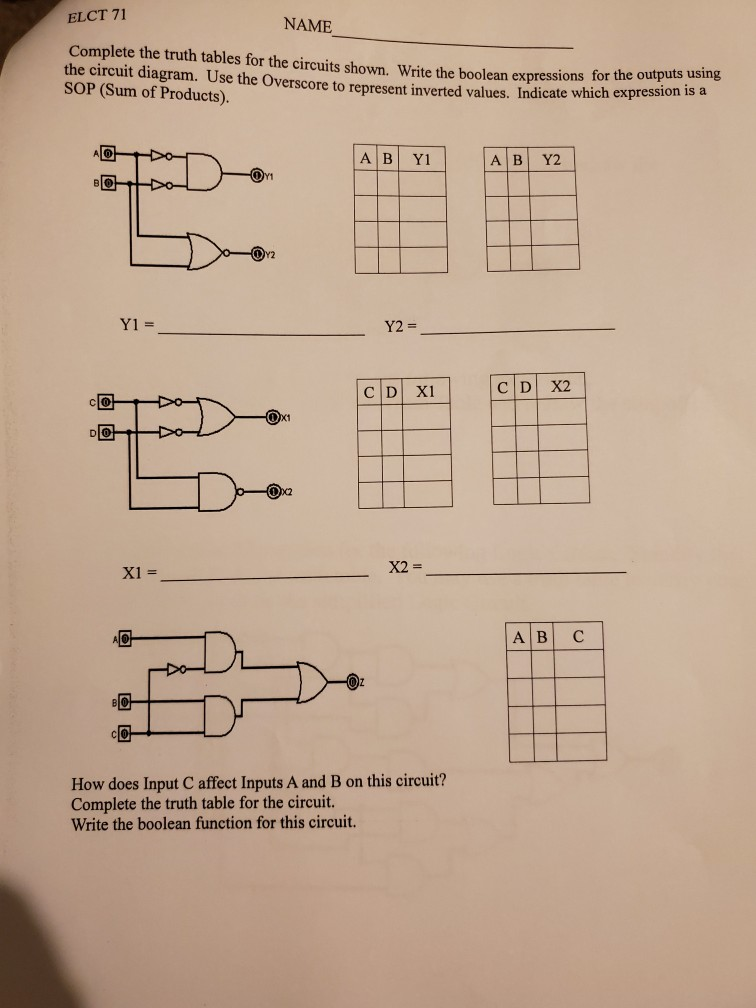# (Solved) : 1 Write Boolean Expression Following Circuit Redraw Equivalent Circuit Using Single Logic Q40048307 . . .1.) Write the Boolean Expression for the following circuit. Redraw the equivalent circuit using a single logic gate. AO 2.) Draw the Digital Logic circuit for the following expression. Simplify the expression. Draw the Truth Table and redraw the simplified circuit. AB.C 3.) Write the Boolean Expression for the following Logic Circuit. Simplify the expression and draw the truth table. You may use a truth table to verify your simplification. Redraw the simplified Logic Circuit. AO 4.) Simplify the following expressions. a.) AB + A(B+C) + B(B+C) b) [ABOC + BD) + A BỆC c.) ĀB C + ABC ABC + AB C + ABC 5.) Using the following circuit: or a.) Write the boolean expression for the circuit. b.) Manipulate the Boolean expression using boolean algebra so it only uses AND, OR and NOT gates. Simplify as much as possible. C.) Write the simplified Boolean expression that produces the inverted output of the circuit. 6.) Write the following expression using OverScore notation and simplify as much as possible. Example: !A = Ā !AB = ĀB !(AB) = AB !A!B = ĀB Q = !(!(A + !B) !(A . !B)) + !A.B 7.) Write the following expression using OverScore notation and simplify as much as possible. Q = !(!(!(A . !C) • !B). D) + !(!C.D) http://sandbox.mc.edu/~bennet/cs110/boolalg/simple.html ELCT 71 NAME Complete the truth tables for the circuits shown. Write the boolean expressions for the circuit diagram. Use the Overscore to represent inverted values. Indicate w SOP (Sum of Products). 1. Write the boolean expressions for the outputs using ted values. Indicate which expression is a AB Y1 AB 72 – Y2 Y1 = _ Y2 = CD xı | X2 X2 C How does Input C affect Inputs A and B on this circuit? Complete the truth table for the circuit. Write the boolean function for this circuit. Show transcribed image text 1.) Write the Boolean Expression for the following circuit. Redraw the equivalent circuit using a single logic gate. AO 2.) Draw the Digital Logic circuit for the following expression. Simplify the expression. Draw the Truth Table and redraw the simplified circuit. AB.C 3.) Write the Boolean Expression for the following Logic Circuit. Simplify the expression and draw the truth table. You may use a truth table to verify your simplification. Redraw the simplified Logic Circuit. AO
4.) Simplify the following expressions. a.) AB + A(B+C) + B(B+C) b) [ABOC + BD) + A BỆC c.) ĀB C + ABC ABC + AB C + ABC 5.) Using the following circuit: or a.) Write the boolean expression for the circuit. b.) Manipulate the Boolean expression using boolean algebra so it only uses AND, OR and NOT gates. Simplify as much as possible. C.) Write the simplified Boolean expression that produces the inverted output of the circuit.
6.) Write the following expression using OverScore notation and simplify as much as possible. Example: !A = Ā !AB = ĀB !(AB) = AB !A!B = ĀB Q = !(!(A + !B) !(A . !B)) + !A.B 7.) Write the following expression using OverScore notation and simplify as much as possible. Q = !(!(!(A . !C) • !B). D) + !(!C.D) http://sandbox.mc.edu/~bennet/cs110/boolalg/simple.html
ELCT 71 NAME Complete the truth tables for the circuits shown. Write the boolean expressions for the circuit diagram. Use the Overscore to represent inverted values. Indicate w SOP (Sum of Products). 1. Write the boolean expressions for the outputs using ted values. Indicate which expression is a AB Y1 AB 72 – Y2 Y1 = _ Y2 = CD xı | X2 X2 C How does Input C affect Inputs A and B on this circuit? Complete the truth table for the circuit. Write the boolean function for this circuit.

Answer to 1.) Write the Boolean Expression for the following circuit. Redraw the equivalent circuit using a single logic gate. AO …

We are the best freelance writing portal. Looking for online writing, editing or proofreading jobs? We have plenty of writing assignments to handle.